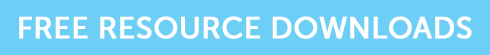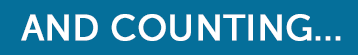How to Calculate Modified Duration

# How to Calculate Modified Duration

Fixed interest securities are also known as bonds, loan stock and even debt securities.  In simpler terms, we know them as gilts and corporate bonds. This article explains the differences in their associated risk and shows how to calculate modified duration. Those who are preparing for any of the CII AF4, R02 or J10 exams will find this useful.

Gilts are considered to be less risky than corporate bonds and both are considered less risky than investing in shares although fixed interest securities are more likely than shares to be affected by interest rates and inflation.

Here we consider the risk of interest rate changes to bond values.

### What is bond duration?

There’s an inverse relationship with bond prices and interest rates; as interest rates fall, bond prices go up and when interest rates go up bond prices fall.  The concept of duration enables an investor to gauge how much a bond’s price is likely to rise or fall when these changes happen.

Duration measures how long it takes in years for the price of the bond to be repaid by its cash flows.  Let’s say this has been calculated as 3.41 years.  We can use this figure and the gross redemption yield to then calculate its modified duration.  Let’s assume the gross redemption yield is 4%.

### How to Calculate Modified Duration

The formula for calculating modified duration is:

Duration of 3.41 divided by (1 + the gross redemption yield of 0.04) = 3.28.

We now know this bond has a modified duration of 3.28 and so can be expected to undergo a 3.28% movement in price for each 1% movement in interest rates.

If the bond had a current price of £108.28 and interest rates rise by 1% then we can expect the bond price to fall to:

£108.28 – (£108.28 x 0.0328) = £104.73

The longer the modified duration is (in years) the more sensitive a bond is to changes in its yield.

### Grab the resources you need!

If you’re studying for your CII R02 exam, and you’re seeking some extra practice, grab our free taster to try out one of Brand Financial Training’s resources for yourself.  Click the link to download the R02 calculation workbook taster now!Alternatively, you can download taster resources for AF4, or J10 if you’re revising for one of those exams.